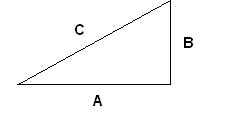# 2. Maths Pythagoras Theorem

Most people who have done maths in high school, would have heard of Pythagoras theorem.

This is used to work out the unknown side of a triangle, if you know the other 2 sides.Here you can see the sides, A, B and C.

Now Pythagoras theorem states that A^2 + B^2 = C^2

So if you know any two sides, you add them in, rearrange the formula if needed, and then you can solve for the unkown sides.

So how would you use this in a game? What about collision detection? Using this you can find quite easily in a 2 dimensional plane when 2 points will meet.

They will meet when A^2 + B^2 = 0

Quite simple really, you take the position of A and B on the X axis and do the equation, then if it comes up with an answer of 0, you then take the position of A and B on the Y axis, then if that also comes to 0, they must be colliding.

So to put that in other words, we are finding the distance 🙂 If the distance is nothing, then they are located in the same position.

Not sure if that is right, sounds right from my position, although it is 1:18 am.

If you have any questions, please email me at swiftless@gmail.com

• March 25, 2010
• 4